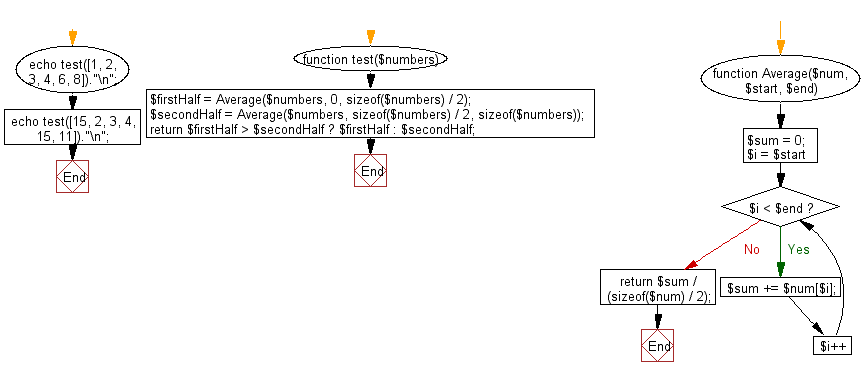﻿ PHP Exercises: Find the larger average value between the first and the second half of a given array of integers and minimum length is atleast 2 - w3resource# PHP Exercises: Find the larger average value between the first and the second half of a given array of integers and minimum length is atleast 2

## PHP Basic Algorithm: Exercise-132 with Solution

Write a PHP program to find the larger average value between the first and the second half of a given array of integers and minimum length is atleast 2. Assume that the second half begins at index (array length)/2.

Sample Solution:

PHP Code :

``````<?php
function test(\$numbers)
{
\$firstHalf = Average(\$numbers, 0, sizeof(\$numbers) / 2);
\$secondHalf = Average(\$numbers, sizeof(\$numbers) / 2, sizeof(\$numbers));
return \$firstHalf > \$secondHalf ? \$firstHalf : \$secondHalf;
}

function Average(\$num, \$start, \$end)
{
\$sum = 0;
for (\$i = \$start; \$i < \$end; \$i++)
\$sum += \$num[\$i];
return \$sum / (sizeof(\$num) / 2);
}

echo test([1, 2, 3, 4, 6, 8])."\n";
echo test([15, 2, 3, 4, 15, 11])."\n";
``````

Sample Output:

```6
10
```

Flowchart:PHP Code Editor:

What is the difficulty level of this exercise?

﻿

## PHP: Tips of the Day

\$_REQUEST: This SuperGlobal Variable is used to collect data submitted by a HTML Form

Example:

This code save in a php file and run in the browser.

```<!DOCTYPE html>
<html>
<body>

<form method="post" action="<?php echo \$_SERVER['PHP_SELF'];?>">
NAME: <input type="text" name="user">
<button type="submit">SUBMIT</button>
</form>
<?php
if (\$_SERVER["REQUEST_METHOD"] == "POST") {
\$name = htmlspecialchars(\$_REQUEST['user']);
if(empty(\$name)){
echo "Name is empty";
} else {
echo \$name;
}
}
?>
</body>
</html>
```

Output:

```Owen
```